The Lewis Definitions of Acids and Bases

The Lewis Definitions of Acids and Bases

In 1923 G. N. Lewis suggested another way of looking at the reaction between H+ and OH- ions. In the Brnsted model, the OH- ion is the active species in this reactionit accepts an H+ ion to form a covalent bond. In the Lewis model, the H+ ion is the active speciesit accepts a pair of electrons from the OH- ion to form a covalent bond.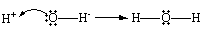In the Lewis theory of acid-base reactions, bases donate pairs of electrons and acids accept pairs of electrons. A Lewis acid is therefore any substance, such as the H+ ion, that can accept a pair of nonbonding electrons. In other words, a Lewis acid is an electron-pair acceptor. A Lewis base is any substance, such as the OH- ion, that can donate a pair of nonbonding electrons. A Lewis base is therefore an electron-pair donor.

One advantage of the Lewis theory is the way it complements the model of oxidation-reduction reactions. Oxidation-reduction reactions involve a transfer of electrons from one atom to another, with a net change in the oxidation number of one or more atoms.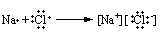The Lewis theory suggests that acids react with bases to share a pair of electrons, with no change in the oxidation numbers of any atoms. Many chemical reactions can be sorted into one or the other of these classes. Either electrons are transferred from one atom to another, or the atoms come together to share a pair of electrons.

The principal advantage of the Lewis theory is the way it expands the number of acids and therefore the number of acid-base reactions. In the Lewis theory, an acid is any ion or molecule that can accept a pair of nonbonding valence electrons. In the preceding section, we concluded that Al3+ ions form bonds to six water molecules to give a complex ion.

Al3+(aq) + 6 H2O(l)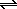Al(H2O)63+(aq)

This is an example of a Lewis acid-base reaction. The Lewis structure of water suggests that this molecule has nonbonding pairs of valence electrons and can therefore act as a Lewis base. The electron configuration of the Al3+ ion suggests that this ion has empty 3s, 3p, and 3d orbitals that can be used to hold pairs of nonbonding electrons donated by neighboring water molecules.

Al3+ = [Ne] 3s0 3p0 3d0

Thus, the Al(H2O)63+ ion is formed when an Al3+ ion acting as a Lewis acid picks up six pairs of electrons from neighboring water molecules acting as Lewis bases to give an acid-base complex, or complex ion.

The Lewis acid-base theroy explains why BF3 reacts with ammonia. BF3 is a trigonal-planar molecule because electrons can be found in only three places in the valence shell of the boron atom. As a result, the boron atom is sp2 hybridized, which leaves an empty 2pz orbital on the boron atom. BF3 can therefore act as an electron-pair acceptor, or Lewis acid. It can use the empty 2pz orbital to pick up a pair of nonbonding electrons from a Lewis base to form a covalent bond. BF3 therefore reacts with Lewis bases such as NH3 to form acid-base complexes in which all of the atoms have a filled shell of valence electrons, as shown in the figure below.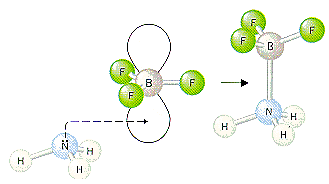The Lewis acid-base theory can also be used to explain why nonmetal oxides such as CO2 dissolve in water to form acids, such as carbonic acid H2CO3.

CO2(g) + H2O(l)H2CO3(aq)

In the course of this reaction, the water molecule acts as an electron-pair donor, or Lewis base. The electron-pair acceptor is the carbon atom in CO2. When the carbon atom picks up a pair of electrons from the water molecule, it no longer needs to form double bonds with both of the other oxygen atoms as shown in the figure below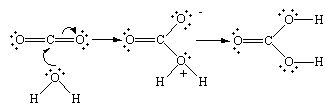One of the oxygen atoms in the intermediate formed when water is added to CO2 carries a positive charge; another carries a negative charge. After an H+ ion has been transferred from one of these oxygen atoms to the other, all of the oxygen atoms in the compound are electrically neutral. The net result of the reaction between CO2 and water is therefore carbonic acid, H2CO3.

 Practice Problem 9:Predict whether the following ions or molecules can act as either a Lewis acid or a Lewis base. (a) Ag+ (b) NH3 Click here to check your answer to Practice Problem 9